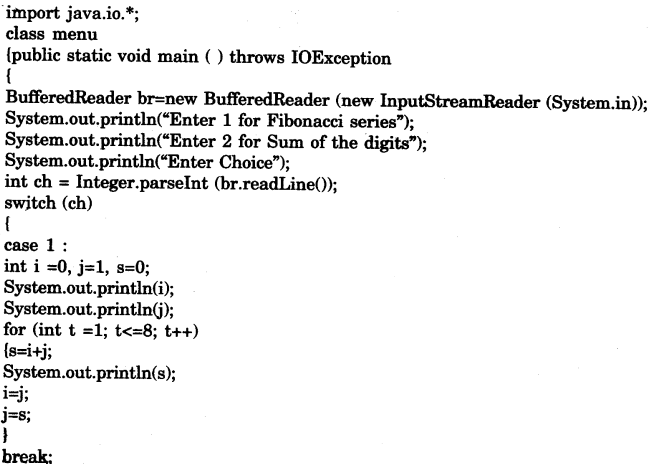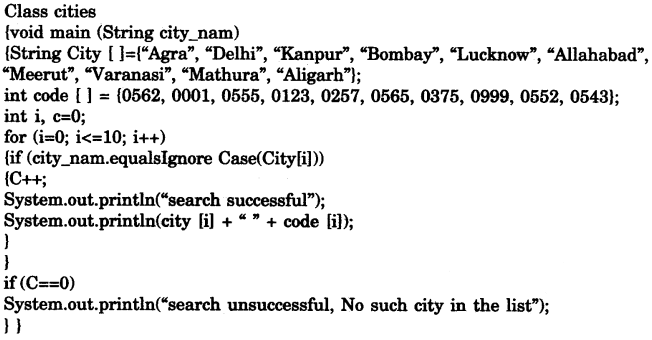## ICSE Computer Applications Previous Year Question Paper 2012 Solved for Class 10

ICSE Paper 2012
COMPUTER APPLICATIONS

(Two Hours)
Answers to this Paper must be written on the paper provided separately.
You will not be allowed to write during the first 15 minutes.
This time is to be spent in reading the question paper.
The time given at the head of this Paper is the time allowed for writing the answers.
This paper is divided into two Sections.
Attempt all questions from Section A and any four questions from Section B.
The intended marks for questions or parts of questions are given in brackets [ ].

Section ‘A’ (40 Marks)

(Attempt all questions)

Question 1:
(a) Give one example each of a primitive data type and a composite data type. 
(b) Give one point of difference between unary and binary operators. 
(c) Differentiate between call by value or pass by value and call by reference or pass by reference. 
(d) Write a Java expression for $$\sqrt { 2as+{ u }^{ 2 } }$$ 
(e) Name the type of error (syntax, runtime or logical error) in each case given below :
(i) Division by a variable that contains a value of zero.
(ii) Multiplication operator used when the operation should be division.
(iii) Missing semicolon. 

(a) Primitive data type: int
Composite data type: Class

(b) Unary operators work on a single operand.
Binary operators act on two operands.

(c) Call by Value: Actual parameters are copied into the formal parameters and the called method works with the formal parameters. Any change in the formal parameter is not reflected back in the actual parameter.
Call by reference: When a method is called, the reference/address on which the data is stored is sent to the formal parameters. Any change in formal parameters is reflected in the actual parameters.

(d) Math.sqrt((2*a*s) + u*u);

(e) (i) runtime error.
(ii) logical error
(iii) Syntax error

Question 2:
(a) Create a class with one integer instance variable. Initialize the variable using:
(i) default constructor.
(ii) parameterized constructor. 
(b) Complete the code below to create an object of Scanner class :
Scanner sc = ………. Scanner (………). 
(c) What is an array ? Write a statement to declare an integer array of 10 elements. 
(d) Name the search or sort algorithm that :
(i) Makes several passes through the array, selecting the next smallest item in the array each time and placing it where it belongs in the array.
(ii) At each stage, compares the sought key value with the key value of the middle element of the array. 
(e) Differentiate between public and private modifiers for members of a class. 

(a) class Test
{ int n;
Test ( )
{n=0; }
Test (int nn)
{n=nn;}
}

(b) Scanner sc = new Scanner (System.in);

(c) An array is a group of similar type variables that are referenced by a common name.
int arr = new int ;

(d) (i) Selection sort
(ii) Binary search

(e) Public Modifiers: Public members of a class are available to all the classes of all the packages.
Private Modifiers: Private members of a class are accessible only within the class.

Question 3:
(a) What are the values of x and y when the following statement are executed ?
int a =63, b=36;
boolean x=(a>b)?true:false;
int y=(a<b)?a:b; 

(b) State the values of n and ch.
char c=‘A’;
int n=c+1;
char ch=(char)n; 

(c) What will be the result stored in x after evaluating the following expression ?
int x=4; x+=(x++)+(++x)+x; 

(d) Give the output of the following program segment :
double x=2.9, y=2.5;
System.out.println(Math.min(Math.floor(x),y));
System.out.println(Math.max(Math.ceil(x),y)); 

(e) State the output of the following program segment :
String s=“Examination”;
int n=s.length();
System.out.println(s.starts With(s.substring(5,n)));
System.out.println(s.charAt(2)==s.charAt(6)); 

(f) State the method that:
(i) Converts a string to a primitive float data type.
(ii) Determines if the specified character is an uppercase character. 

(g) State the data type and values of a and b after the following segment is executed.
String s1=“Computer”, s2=“Applications”;
a=(s1.compareTo(s2));
b=(s1.equals(s2)); 

(h) What will the following code output ?
String s=“malayalam”;
System.out.println(s.indexOf(‘m’));
System.out.println(s.lastIndexOf(‘m’)); 

(i) Rewrite the following program segment using while instead of for statement,
int f=1,i;
for(i=1; i<=5; i++)
(f*=i; System.out.println(f);} 

(j) In the program given below, state the name and the value of the
(i) method argument or argument variable
(ii) class variable
(iii) local variable
(iv) instance variable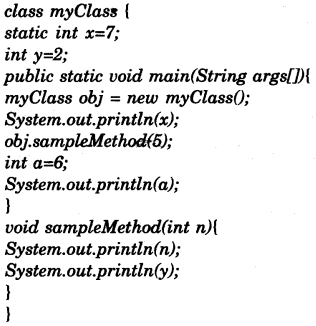(a) x = true
y = 36

(b) n = 66
ch = B

(c) x = 20

(d) 2.0
3.0

(e) False True.

(f) (i) Float.valueOf (<string>);
(ii) Character.isUpperCase (<character>);

(g) int, a = 2
boolean, b = false

(h) 0
8

(i) int f = 1, i = 1;
while (i < = 5)
{ f* = i;
System.out.println(f);
i++; }

(j) (i) n
(ii) x
(iii) a
(iv) y

SECTION B (60 Marks)

Attempt any four questions from this Section.
The answers in this Section should consist of the Programs in either Blue J environment or any program environment with Java as the base.
Each program should be written using Variable descriptions /Mnemonic Codes so that the logic of the program is clearly depicted.
Flow-Charts and Algorithms are not required.

Question 4:
Define a class called Library with the following description :
Instance variables/data members :
int acc_num — stores the accession number of the book
String title — stores the title of the book
String author — stores the name of the author
Member methods:
(i) void input ( ) — To input and store the accession number, title and author.
(ii) void compute ( ) — To accept the number of days late, calculate the display the fine charged at the rate of Rs. 2 per day.
(iii) void display( ) — To display the details in the following format:
Accession      Number      Title Author
Write a main method to create an object of the class and call the above member methods.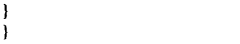Question 5:
Given below is a hypothetical table showing rates of Income Tax for male citizens below the age of 65 years :

 Taxable Income (TI) in Rs. Income Tax in Rs. Does not exceed Rs. 1,60,000 Nil Is greater than Rs. 1,60,000 and less than or equal to Rs. 5,00,000 (TI—1,60,000) × 10% Is greater than Rs. 5,00,000 and less than or equal to Rs. 8,00,000 [(TI—5,00,000 × 20%] + 34,000 Is greater than Rs. 8,00,000 [(TI—8,00,000 × 30%] + 94,000

Write a program to input the age, gender (male or female) and Taxable Income of a person.
If the age is more than 65 years or the gender is female, display “wrong category”.
If the age is less than or equal to 65 years and the gender is male, compute and display the Income Tax payable as per the table given above.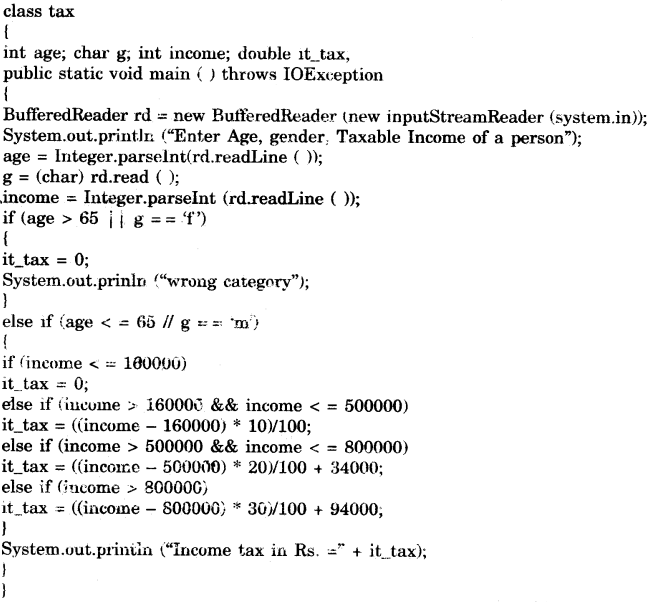Question 6:
Write a program to accept a string. Convert the string to uppercase. Count and output the number of double letter sequences that exist in the string.
Sample Input: “SHE WAS FEEDING THE LITTLE RABBIT WITH AN APPLE”
Sample Output: 4Question 7:
Design a class to overload a function polygon() as follows
(i) void polygon(int n, char ch) — with one integer argument and one character type argument that draws a filled square of side n using the character stored in ch.
(ii) void polygon(int x, int y) — with two integer arguments that draws a filled rectangle of length x and breadth y, using the symbol ‘@’.
(iii) void polygon( ) — with no argument that draws a filled an angle shown below.
Example :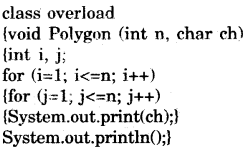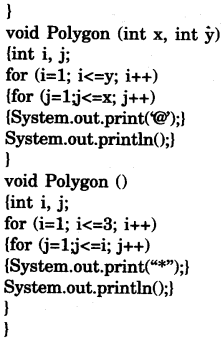Question 8:
Using the switch statement, write a menu driven program to :
(i) Generate and display the first 10 terms of the Fibonacci series 0, 1, 1, 2, 3, 5…..
The first two Fibonacci numbers are 0 and 1, and each subsequent number is the sum of the previous two.
(ii) Find the sum of the digits of an integer that is input.
Sample Input: 15390
Sample Output : Sum of the digits =18
For an incorrect choice, an appropriate error message should be displayed.